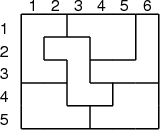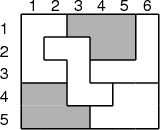# Internet Problem Solving Contest

## Problem Q – Quick search

The second problem in this practice session is based on an actual pen and paper puzzle. The puzzle, as you’ll soon discover, is quite tricky – and harder than it might seem.

Problem specification

In this task you will be given a rectangle divided into several polygons. All of these polygons are distinct, except for two that are identical. Your task is to find the two identical ones.

Note: We call two polygons identical iff one of them can be obtained from the other by a combination of translations and rotations. Note that it is not allowed to use axis symmetry – i.e., if a polygon is just a mirror image of another one, they are considered distinct for the purpose of this problem.

Input specification

The input is an image of the rectangle, including labels for rows and columns.

Output specification

Each polygon in the input is uniquely identified by the coordinates (r,c) of its upper left corner. More precisely, to describe a polygon, we first find the smallest row r in which the polygon contains at least one cell, and then pick the smallest possible column c it contains in this row.

Once you locate the two polygons, output the coordinates (r1,c1) and (r2,c2) of their upper left corners.

To make the output unique, we require that r1 r2, and if r1 = r2, then c1 < c2. I.e., first output the polygon that starts earlier.

Example

 inputoutput 1 3  4 1Note that the polygon at (4,5) is not identical to the highlighted pair.